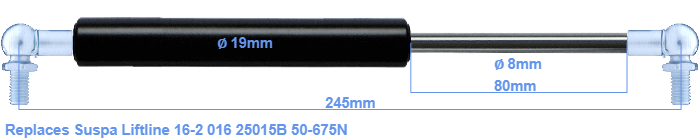# Replacement for Suspa Liftline 16-2 016 25015B 50-675N

\$35.06

Replacement gas spring for the Suspa Liftline 16-2 016 25015B 50-675 Newton. This fits a (possibly already present) ball with a diameter of 10mm. A corresponding ball is included. Brand: Stabilus Industry Line.
 Force Choose an option50 Newton60 Newton80 Newton100 Newton120 Newton140 Newton150 Newton160 Newton180 Newton200 Newton220 Newton240 Newton250 Newton260 Newton280 Newton300 Newton320 Newton340 Newton350 Newton360 Newton380 Newton400 Newton420 Newton440 Newton450 Newton460 Newton480 Newton500 Newton520 Newton540 Newton550 Newton560 Newton580 Newton600 Newton620 Newton640 Newton650 Newton660 Newton675 NewtonClearThe diameter of the black cylinder of this gas spring is 19 millimeter. The rod has a diameter of 8 mm. The stroke is 80 millimeter long. The total length of this replacement is 245 millimeter. Keep in mind that this is the length between the centers of the mounting parts. Without the mounting parts this gas spring is 205 mm long (thread to thread). The force of this replacement gas spring is 50-675 Newton. This gas spring can replace a Suspa Liftline gas spring, but is not an orignal Suspa Liftline one. This gas spring was produced by Stabilus subsidiary Hahn with the brand Stabilus Industry Line. Nevertheless, the dimensions and force are equal.
Category: